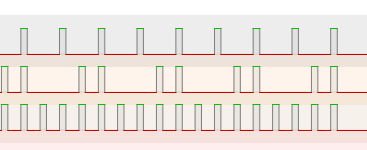# How to compare to last value

I’m using a Bela and connecting ESP32 GPIOs to the Bela digital ins. I have a simple ESP32 program that counts which results in lighting up the connected GPIO pins which is detected by a SynthDef. I works great, and I can visually inspect it counting as I use SentTrig to send the value.

I want to increase the frequency of the test to see how fast it can go, while stressing some other things so I want to have it produce a message when the current value is not 1 more than the previous value. That way I’ll know it lost one or more values because it will be impossible to use my eyes to see it working. I get confused with state and sclang and I’m wondering if somebody can help me accomplish this type of test to verify the values are continually incrementing without a gap?

``````var digitalPins = [0, 1, 2, 3, 4];

SynthDef('sh', {
var trigger;

// Create an array to store the digital input signals.
var d = Array.fill(digitalPins.size, { |i|
DigitalIn.ar(digitalPins[i]);
});

// Convert the bit array to a binary value
trigger = d.reverse.reduce({ arg total, bit; total * 2 + bit }, 0);
SendTrig.ar(TDelay.ar(in: trigger, dur: 0.001), 0, trigger);

}).send(s);

s.sync;
Synth.new("sh");

OSCFunc({ arg msg, time; [time, msg].postln; },'/tr', a);
``````

Thanks, Paul

For signals, you can think of comparison as subtraction:

• If a - b > 0, then a > b.
• If a - b == 0, then a == b.
• If a - b < 0, then a < b.

So then you’re looking for something that will subtract the previous sample value from the current one: current - last.

This is HPZ1: “A special case fixed filter. Implements the formula: out(i) = 0.5 * (in(i) - in(i-1)).”

If the signal is not changing most of the time, then HPZ1 outputs 0.

You’re looking for current - last > 1 – accounting for the 0.5 coefficients, then 0.5 * (current - last) > 0.5.

``````SendTrig.ar(HPZ1.ar(trigger) > 0.5, ...)
``````

(Note that “state and sclang” aren’t relevant because you’re working with signals.)

hjh

That is very helpful, thanks @jamshark70 . But since I’m setting the digital lines briefly, and then back to zero the trigger, which I think I understand is a signal, it could have a series of values like 5, 0, 0, 6, 0, 0, 0, 7, 0, 0, and so on. So, I need another thing (ugen?) that only remembers the nonzero values?

If it helps, a logic analyzer shows:This is Latch, using the signal as both the input and the trigger.

hjh

Thanks so much @jamshark70. Here’s the final code that works for me:

``````// Replace with the actual pin numbers.
var digitalPins = [0, 1, 2, 3, 4, 5, 6, 11, 12, 7, 8, 9];

// Create a variable to store the last computed value
~lastValue = 4;

SynthDef('sh', {
var trigger, delay_trigger, trigSig, lastTrig;

// Create an array to store the digital input signals.
var d = Array.fill(digitalPins.size, { |i|
DigitalIn.ar(digitalPins[i]);
});

// Convert the bit array to a binary value
trigger = d.reverse.reduce({ arg total, bit; total * 2 + bit }, 0);
delay_trigger = TDelay.ar(in: trigger, dur: 0.001);

//SendTrig.ar(delay_trigger, 0, trigger);
SendTrig.ar(HPZ1.ar(Latch.ar(trigger, delay_trigger)) > 0.5, 1, trigger);
}).send(s);

s.sync;
Synth.new("sh");

OSCFunc({ arg msg, time; [time, msg].postln; },'/tr', a);
``````